# Escape velocity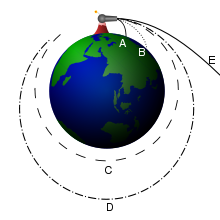Isaac Newton's analysis of escape velocity. Projectiles A and B fall back to earth. Projectile C achieves a circular orbit, D an elliptical one. Projectile E escapes.

In physics, escape velocity is the speed of an object at which its kinetic energy is equal to the magnitude of its gravitational potential energy, as calculated by the equation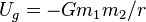$U_g = -Gm_1m_2/r$. It is commonly described as the speed needed to "break free" from a gravitational field (without any additional impulse). The term escape velocity actually refers to a speed rather than a velocity—that is, it specifies how fast the object must move, but the direction of movement is irrelevant. In more technical terms, escape velocity is a scalar (not a vector) quantity.

## Overview

The phenomenon of escape velocity is a consequence of conservation of energy. For an object with a given total energy, which is moving subject to conservative forces (such as a static gravity fields) the object can reach only combinations of places and speeds which have that total energy; and places which have a higher potential energy than this cannot be reached at all.

For a given gravitational potential energy at a given position, the escape velocity is the minimum speed an object without propulsion needs to have sufficient energy to be able to "escape" from the gravity, that is, so that gravity will never manage to pull it back. For the sake of simplicity, unless stated otherwise, this article will assume that the scenario one is dealing with is that an object is attempting to escape from a uniform spherical planet by moving straight up (along a radial line away from the center of the planet), and that the only significant force acting on the moving object is the planet's gravity.

Escape velocity is actually a speed (not a velocity) because it does not specify a direction: no matter what the direction of travel is, the object can escape the gravitational field. The simplest way of deriving the formula for escape velocity is to use conservation of energy. Imagine that a spaceship of mass m is at a distance r from the center of mass of the planet, whose mass is M. Its initial speed is equal to its escape velocity,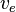$v_e$. At its final state, it will be an infinite distance away from the planet, and its speed will be negligibly small and assumed to be 0. Kinetic energy K and gravitational potential energy Ug are the only types of energy that we will deal with, so by the conservation of energy,$(K + U_g)_i = (K + U_g)_f. \,$

Kf = 0 because final velocity is zero, and Ugf = 0 because its final distance is infinity, so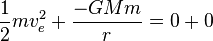$\frac{1}{2}mv_e^2 + \frac{-GMm}{r} = 0 + 0$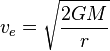$v_e = \sqrt{\frac{2GM}{r}}$

Defined a little more formally, "escape velocity" is the initial speed required to go from an initial point in a gravitational potential field to infinity with a residual velocity of zero, with all speeds and velocities measured with respect to the field. Additionally, the escape velocity at a point in space is equal to the speed that an object would have if it started at rest from an infinite distance and was pulled by gravity to that point. In common usage, the initial point is on the surface of a planet or moon. On the surface of the Earth, the escape velocity is about 11.2 kilometers per second (~6.96 mi/s), which is approximately 34 times the speed of sound (mach 34) and at least 10 times the speed of a rifle bullet. However, at 9,000 km altitude in "space," it is slightly less than 7.1 km/s.

The escape velocity relative to the surface of a rotating body depends on direction in which the escaping body travels. For example, as the Earth's rotational velocity is 465 m/s at the equator, a rocket launched tangentially from the Earth's equator to the east requires an initial velocity of about 10.735 km/s relative to Earth to escape whereas a rocket launched tangentially from the Earth's equator to the west requires an initial velocity of about 11.665 km/s relative to Earth. The surface velocity decreases with the cosine of the geographic latitude, so space launch facilities are often located as close to the equator as feasible, for example, the American Cape Canaveral (latitude 28°28' N) and the French Guiana Space Centre (latitude 5°14' N).

Escape velocity is independent of the mass of the escaping object. It does not matter if the mass is 1 kg or 1000 kg, escape velocity from the same point in the same gravitational field is always the same. What differs is the amount of energy needed to accelerate the mass to achieve escape velocity: The energy needed for an object of mass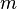$m$ to escape the Earth's gravitational field is GMm / r, a function of the object's mass (where r is the radius of the Earth, G is the gravitational constant, and M is the mass of the Earth). More massive objects require more energy to reach escape velocity. All of this, of course, assumes one is neglecting air resistance.

## Misconceptions

Planetary or lunar escape velocity is sometimes misunderstood to be the speed a powered vehicle (such as a rocket) must reach to leave orbit; however, this is not the case, as the quoted number is typically the surface escape velocity, and vehicles never achieve that speed direct from the surface. This surface escape velocity is the speed required for an object to leave the planet if the object is simply projected from the surface of the planet and then left without any more kinetic energy input: In practice the vehicle's propulsion system will continue to provide energy after it has left the surface.

In fact a vehicle can leave the Earth's gravity at any speed. At higher altitude, the local escape velocity is lower. But at the instant the propulsion stops, the vehicle can only escape if its speed is greater than or equal to the local escape velocity at that position. At sufficiently high altitude this speed can approach 0.

## Orbit

If an object attains escape velocity, but is not directed straight away from the planet, then it will follow a curved path. Even though this path will not form a closed shape, it is still considered an orbit. Assuming that gravity is the only significant force in the system, this object's speed at any point in the orbit will be equal to the escape velocity at that point (due to the conservation of energy, its total energy must always be 0, which implies that it always has escape velocity; see the derivation above). The shape of the orbit will be a parabola whose focus is located at the center of mass of the planet. An actual escape requires of course that the orbit not intersect the planet, since this would cause the object to crash. When moving away from the source, this path is called an escape orbit; when moving closer to the source, a capture orbit. Both are known as C3 = 0 orbits (where C3 = - μ/a, and a is the semi-major axis).

Remember that in reality there are many gravitating bodies in space, so that, for instance, a rocket that travels at escape velocity from Earth will not escape to an infinite distance away because it needs an even higher speed to escape the Sun's gravity. In other words, near the Earth, the rocket's orbit will appear parabolic, but eventually its orbit will become an ellipse around the Sun.

## List of escape velocitiesTo leave planet Earth an escape velocity of 11.2 km/s is required, however a speed of 42.1 km/s is required to escape the Sun's gravity (and exit the solar system) from the same position
Location with respect to Ve     Location with respect to Ve
on the Sun, the Sun's gravity: 617.5 km/s
on Mercury, Mercury's gravity: 4.4 km/s at Mercury, the Sun's gravity: 67.7 km/s
on Venus, Venus' gravity: 10.4 km/s at Venus, the Sun's gravity: 49.5 km/s
on Earth, the Earth's gravity: 11.2 km/s at the Earth/Moon, the Sun's gravity: 42.1 km/s
on the Moon, the Moon's gravity: 2.4 km/s at the Moon, the Earth's gravity: 1.4 km/s
on Mars, Mars' gravity: 5.0 km/s at Mars, the Sun's gravity: 34.1 km/s
on Jupiter, Jupiter's gravity: 59.5 km/s at Jupiter, the Sun's gravity: 18.5 km/s
on Saturn, Saturn's gravity: 35.5 km/s at Saturn, the Sun's gravity: 13.6 km/s
on Uranus, Uranus' gravity: 21.3 km/s at Uranus, the Sun's gravity: 9.6 km/s
on Neptune, Neptune's gravity: 23.5 km/s at Neptune, the Sun's gravity: 7.7 km/s
in the solar system,   the Milky Way's gravity:   ~1,000 km/s

Because of the atmosphere it is not useful and hardly possible to give an object near the surface of the Earth a speed of 11.2 km/s, as these speeds are too far in the hypersonic regime for most practical propulsion systems and would cause most objects to burn up due to atmospheric friction. For an actual escape orbit a spacecraft is first placed in low Earth orbit and then accelerated to the escape velocity at that altitude, which is a little less—about 10.9 km/s. The required acceleration, however, is generally even less because from that sort of an orbit the spacecraft already has a speed of 8 km/s.

## Calculating escape velocity

To expand upon the derivation given in the Overview,$v_e = \sqrt{\frac{2GM}{r}} = \sqrt{\frac{2\mu}{r}} = \sqrt{2gr\,}.$

where$v_e$ is the escape velocity, G is the gravitational constant, M is the mass of the body being escaped from, m is the mass of the escaping body, r is the distance between the center of the body and the point at which escape velocity is being calculated, g is the gravitational acceleration at that distance, and μ is the standard gravitational parameter.

The escape velocity at a given height is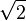$\sqrt 2$ times the speed in a circular orbit at the same height (compare this with equation (14) in circular motion). This corresponds to the fact that the potential energy with respect to infinity of an object in such an orbit is minus two times its kinetic energy, while to escape the sum of potential and kinetic energy needs to be at least zero.

For a body with a spherically-symmetric distribution of mass, the escape velocity$v_e$ from the surface (in m/s) is approximately 2.364×10−5 m1.5kg−0.5s−1 times the radius r (in meters) times the square root of the average density ρ (in kg/m³), or:$v_e \approx 2.364 \times 10^{-5} r \sqrt \rho.\,$

## Deriving escape velocity using calculus

These derivations use calculus, Newton's laws of motion and Newton's law of universal gravitation.

### Derivation using only g and r

The Earth's escape speed can be derived from "g," the acceleration due to gravity at the Earth's surface. It is not necessary to know the gravitational constant G or the mass M of the Earth. Let

r = the Earth's radius, and
g = the acceleration of gravity at the Earth's surface.

Above the Earth's surface, the acceleration of gravity is governed by Newton's inverse-square law of universal gravitation. Accordingly, the acceleration of gravity at height s above the center of the Earth (where s > r ) is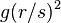$g (r / s)^2$. The weight of an object of mass m at the surface is g m, and its weight at height s above the center of the Earth is gm (r / s)². Consequently the energy needed to lift an object of mass m from height s above the Earth's center to height s + ds (where ds is an infinitesimal increment of s) is gm (r / sds. Since this decreases sufficiently fast as s increases, the total energy needed to lift the object to infinite height does not diverge to infinity, but converges to a finite amount. That amount is the integral of the expression above: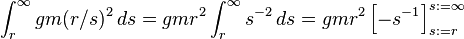$\int_r^\infty gm (r/s)^2 \, ds =gmr^2 \int_r^\infty s^{-2}\,ds =gmr^2 \left[-s^{-1}\right]_{s:=r}^{s:=\infty}$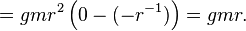$=gmr^2\left(0-(-r^{-1})\right)=gmr.$

That is how much kinetic energy the object of mass m needs in order to escape. The kinetic energy of an object of mass m moving at speed v is (1/2)mv². Thus we need$\begin{matrix}\frac12\end{matrix} mv^2=gmr.$

The factor m cancels out, and solving for v we get$v=\sqrt{2gr\,}.$

If we take the radius of the Earth to be r = 6400 kilometers and the acceleration of gravity at the surface to be g = 9.8 m/s², we get$v\cong\sqrt{2\left(9.8\ {\mathrm{m}/\mathrm{s}^2}\right)(6.4\times 10^6\ \mathrm{m})}= 11\,201\ \mathrm{m}/\mathrm{s}.$

This is just a bit over 11 kilometers per second, or a bit under 7 miles per second, as Isaac Newton calculated.

### Derivation using G and M

Let G be the gravitational constant and let M be the mass of the earth or other body to be escaped.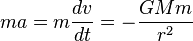$ma=m\frac{dv}{dt}=-\frac{GMm}{r^2}\,$$a=\frac{dv}{dt}=-\frac{GM}{r^2}\,$

By applying the chain rule, one gets:$\frac{dv}{dt}=\frac{dv}{dr} \cdot \frac{dr}{dt}=-\frac{GM}{r^2}\,$

Because$v=\frac{dr}{dt}$$\frac{dv}{dr}\cdot v = -\frac{GM}{r^2}\,$$v \cdot dv = -\frac{GM}{r^2}\,dr\,$$\int_{v_0}^{v(t)} v\,dv = -\int_{r_0}^{r(t)}\frac{GM}{r^2}\,dr\,$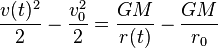$\frac{v(t)^2}{2}-\frac{v_0^2}{2} = \frac{GM}{r(t)}-\frac{GM}{r_0}\,$

Since we want escape velocity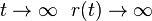$t \rightarrow \infty \ \ r(t) \rightarrow \infty$ and$v(t) \rightarrow 0$$-\frac{v_0^2}{2} = -\frac{GM}{r_0}\,$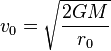$v_0 = \sqrt\frac{2GM}{r_0}\,$

v0 is the escape velocity and r0 is the radius of the planet. Note that the above derivation relies on the equivalence of inertial mass and gravitational mass.

### The derivations are consistent

The gravitational acceleration can be obtained from the gravitational constant G and the mass of Earth M:$g = \frac{GM}{r^2},$

where r is the radius of Earth. Thus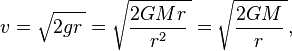$v=\sqrt{2gr\,}=\sqrt{\frac{2GMr}{r^2}\,}=\sqrt{\frac{2GM}{r}\,},$

so the two derivations given above are consistent.

## Multiple sources

The escape velocity from a position in a field with multiple sources is derived from the total potential energy per kg at that position, relative to infinity. The potential energies for all sources can simply be added. For the escape velocity this results in the square root of the sum of the squares of the escape velocities of all sources separately.

For example, at the Earth's surface the escape velocity for the combination Earth and Sun is$\scriptstyle\sqrt{11.2^2\ +\ 42.1^2}\ =\ 43.56\ \mathrm{km}/\mathrm{s}$. As a result, to leave the solar system requires a speed of 13.6 km/s relative to Earth in the direction of the Earth's orbital motion, since the speed is then added to the speed of 30 km/s of that orbital motion

## Gravity well

In the hypothetical case of uniform density, the velocity that an object would achieve when dropped in a hypothetical vacuum hole from the surface of the Earth to the center of the Earth is the escape velocity divided by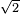$\scriptstyle\sqrt 2$, that is, the speed in a circular orbit at a low height. Correspondingly, the escape velocity from the center of the Earth would be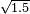$\scriptstyle\sqrt {1.5}$ times that from the surface.

A refined calculation would take into account the fact that the Earth's mass is not uniformly distributed as the center is approached. This gives higher speeds.